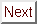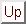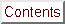# XMM-Newton Science Analysis System: User GuideNext: 6.6.3 Example of grism data processing Up: 6.6 Running the OM data processing Previous: 6.6.1 Example of image data processing

### 6.6.2 Example of fast mode data processing

In a similar way, if an exposure contains fast mode data, the following files will be needed

```0472_0125910501_OMS00400WDX.FIT  - Exposure priority window file
0472_0125910501_OMS00400THX.FIT  - Exposure tracking history file
0472_0125910501_OMS00401FAE.FIT  - Exposure fast mode data file
```

and the process can be run task by task in the following way

```step1> omprep set=0472_0125910501_OMS00400THX.FIT \
nphset=0472_0125910501_OMX00000NPH.FIT \
pehset=0472_0125910501_OMX00000PEH.FIT \
wdxset=0472_0125910501_OMS00400WDX.FIT \
outset=tmp_tracking modeset=3
```

As for image data, if there is no THX file, then set=DUMMYTHX.FIT. omprep will generate a dummy file needed for the rest of the chain, with zero drift in it.

```step2> omdrifthist set=tmp_tracking plotfile=P0125910501OMS004TSHPLT0000.ps

step3> omthconv thxset=tmp_tracking modeset=1 \
nphset=0472_0125910501_OMX00000NPH.FIT \
outset=P0125910501OMS004TSTRTS0000.FIT

step4> omprep set=0472_0125910501_OMS00401FAE.FIT \
nphset=0472_0125910501_OMX00000NPH.FIT \
pehset=0472_0125910501_OMX00000PEH.FIT \
wdxset=0472_0125910501_OMS00400WDX.FIT \
outset=event_list.fit modeset=1
```

In its second run, omprep is invoked for fast data (modeset=1) and the FAE raw event data is transformed into a modified event list to be used as input for evselect

```step5> evselect table=event_list.fit xcolumn=RAWX ycolumn=RAWY \
ximagebinsize=1 yimagebinsize=1 \
withimageset=true imageset=raw_image.fit
```

The pseudo-image corresponding to the fast mode OSW has been created by this first run of evselect.

```step6> omfastshift set=event_list.fit thxset=tmp_tracking \
nphset=0472_0125910501_OMX00000NPH.FIT
```

The X- and Y- coordinates of the photon events are corrected for spacecraft drift. New columns are added to the event list with the corrected values.

```step7> omfastflat set=event_list.fit \
slewflatset=P0125910501OMX000FLAFLD0000.FIT \
oswflatset=fast_flat.fit \
fastimgset=P0125910501OMS002IMAGE_1000.FIT
```

Here again, as for image mode processing, the system is prepared to apply a subset of the omflatgen generated flat field to the fast mode window data, taking into account the spacecraft drift. The flat field is set to one, and therefore this correction has no real effect. The task generates the tracking shifted fast_flat.fit (only for the fast mode window) and the corrected pseudo image image.fit.

```step8 >omdetect set=P0125910501OMS004IMAGE_1000.FIT \
regionfile=region_file nsigma=6 \
outset=P0125910501OMS004SWSRLI1000.FIT
```

The output region will allow the user to check the proper detection of the source in the small fast window. The PSF information is used to parametrise the detected source.

```step9> omatt set=P0125910501OMS004IMAGE_1000.FIT \
sourcelistset=P0125910501OMS004SWSRLI1000.FIT \
ppsoswset=P0125910501OMS004SIMAGE1000.FIT \
usecat=no rotateimage=yes tolerance=3
```

Astrometry is applied as for image data. The pseudo-image is north aligned as well.

```step10> omregion set=P0125910501OMS004SWSRLI1000.FIT \
srcnumber=1 srcradius=-6 nfwhm=3 bkginner=1.2 bkgouter=2.5 \
bkgfile=back_region.fit srcfile=source_region.fit
```

These regions will be used by evselect to filter out the event list and for extracting the corresponding photon events for the source and the background. Optional parameters can be used to fine-tune the definition of the regions.

```step11> evselect table=event_list.fit xcolumn=CORR_X ycolumn=CORR_Y \
expression="((WIN_FLAG .eq. 0) .and. \
(region(source_region.fit, CORR_X, CORR_Y)))" \
maketimecolumn=T withrateset=Y timebinsize=10 \
rateset=source_rate.fit

step12> evselect table=event_list.fit xcolumn=CORR_X ycolumn=CORR_Y \
expression="((WIN_FLAG .eq. 0) .and. \
(region(back_region.fit, CORR_X, CORR_Y)))" \
maketimecolumn=T withrateset=Y timebinsize=10 \
rateset=back_rate.fit
```

And finally the light curve can be obtained and plotted. The photometric corrections are also applied (coincidence loss, dead-time, PSF, magnitude conversion)

```step13> omlcbuild srcregionset=source_region.fit bkgregionset=back_region.fit \
srcrateset=source_rate.fit bkgrateset=back_rate.fit \
sourcelistset=P0125910501OMS004SWSRLI1000.FIT \
wdxset=0472_0125910501_OMS00400WDX.FIT \
outset=P0125910501OMS004TIMESR1000.FIT \
bkgfromimage=yes
```

The optional parameter bkgfromimage, defaulted to yes in the call to omichain, allows the background to be measured in the associated image mode data or in the fast window data.

```step14> lcplot set=P0125910501OMS004TIMESR1000.FIT \
binsize=1 plotdevice=/PS bkgdyscale=no \
plotfile=lightcurve.ps
```Next: 6.6.3 Example of grism data processing Up: 6.6 Running the OM data processing Previous: 6.6.1 Example of image data processing
European Space Agency - XMM-Newton Science Operations Centre